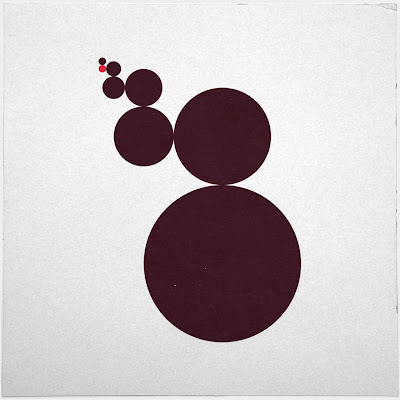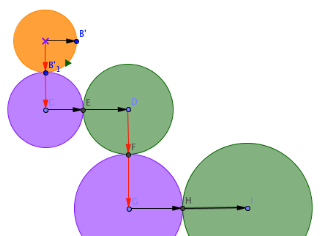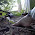## Thursday, April 4, 2013

### Sequential Circular Reasoning

I've been wanting to get better with the Sequence command in GeoGebra. It's a powerful tool for repetitive computation or construction, and math, of course, is full of the patterns. And then I saw...#410 - Eights
From the perpetually fascinating Geometry Daily. Perfect opportunity for Sequencing.

First I dug into the geometry a bit. It seemed to me like the interesting bits were the 90º turns and the constant (looking) increase in scale. That means you could do them as a series of dilations - but it was complicated to think about the centers of dilation. Probably better to just figure out the radius and center of each circle.  It seems important that the circles osculate - the kissing is a big part of the visual effect.

I was also thinking about what you could generalize, as that is the point of making it dynamic.It's also easier to build those things in as sliders at the beginning of a sketch than editing them in later. (Though it's not that much harder.) The angle between circles, the number of circles, the dilation ratio... pretty good start.

To build in the angle I just rotated the the circle defining point by the slider and the opposite of that angle. And then I made vectors in those two directions from the center of the circle.  I was thinking I needed those directions to build the new circles.  The radii of the new circles would just be a, a^2, a^3 if a is the scale.Then came the messy thinking. The center of the first circle is a* the original radius in the direction of one of the points. Then the 2nd circle is.. the 1st radius plus a times that radius in the 2nd direction. I was working it out symbolically, but now that we've got a picture...

So thinking about the centers, I had to organize my data. Usually I scribble on an envelope, but didn't have one handy, so I used Word.
Pretty neat once I sorted it out. I decided to separate the circle centers by the two directions since it was really massy trying to come up with a single sequence to describe the pattern. The key here was the Sequence command.  (Quotes are from the GeoGebra Wiki.)

Sequence[ <Expression>, <Variable i>, <Number a>, <Number b>]
Yields a list of objects created using the given expression and the index i that ranges from number a to number b.
Example:
L = Sequence[(2, i), i, 1, 5] creates a list of points whose y-coordinates range from 1 to 5: L = {(2, 1), (2, 2), (2, 3), (2, 4), (2, 5)}.

A list is what you would expect:
Lists
Using curly braces you can create a list of several objects (e. g. points, segments, circles).
Example:

• L = {A, B, C} gives you a list consisting of three prior defined points A, B, and C.
• L = {(0, 0), (1, 1), (2, 2)} produces a list that consists of the entered points, as well as these nameless points.
Note: By default, the elements of this list are not shown in the Graphics View.

But I couldn't get it to work. I often try to do too much at once, so I decided to make a list of the powers of a, and then the coefficients of u and v. The relevant commands in GeoGebra are:
Element[ <List>, <Number n> ]
Yields the nth element of the list.
Example:
Element[{1, 3, 2}, 2] yields 3, the second element of {1, 3, 2}.
Append[ <List>, >Object> ]
Appends the object to the list.
Example: Append[{1, 2, 3}, 4] creates the list {1, 2, 3, 4}.
Append[ <Object>, <List> ]
Appends the list to the object.
Example: Append[4, {1, 2, 3}] creates the list {4, 1, 2, 3}.
There is also Insert if you need more control than that.

So I defined my lists
• ays = Sequence[a^(i), i, 1, N]
• cofs = Append[0, Sequence[Sum[ays, k], k, 1, Length[ays]]]
It took a little monkeying around to get these next commands to work. I'm not sure if copying from Word was introducing extra characters or what.
• Sequence[B'_1 + Element[cofs, 2n] u + Element[cofs, 2n - 1] v, n, 1, 10] (list1)
• Sequence[B'_1 + Element[cofs, 2n + 1] v + Element[cofs, 2n] u, n, 1, 10] (list2)
Then the circles are easy!
• CirclesList1 = Sequence[Circle[Element[list1, n], r Element[ays, 2n - 1]], n, 1, N]
• CirclesList2 = Sequence[Circle[Element[list2, n], r Element[ays, 2n]], n, 1, N]
I'm pretty happy with the results. If you'd like to play with the result or remix it for yourself, it's on GeoGebraTube for download or as a mobile-ready applet.
Geometry Daily is a good source for GeoGebra inspiration, as well as good geometry, and just beautiful art. (Usually I put them on my Tumblr.)

1.2.